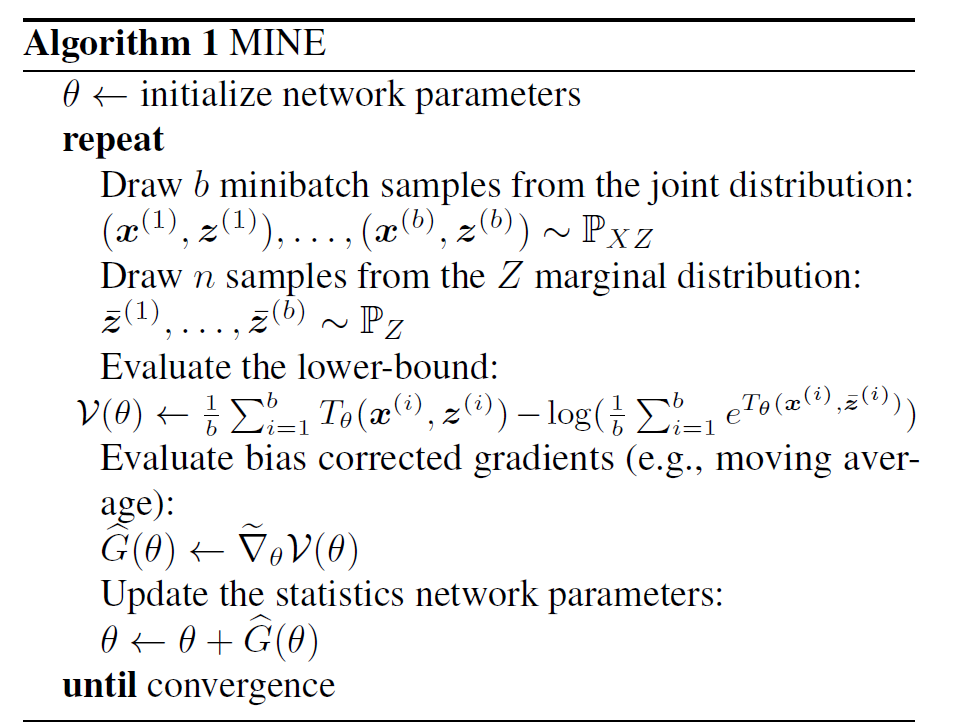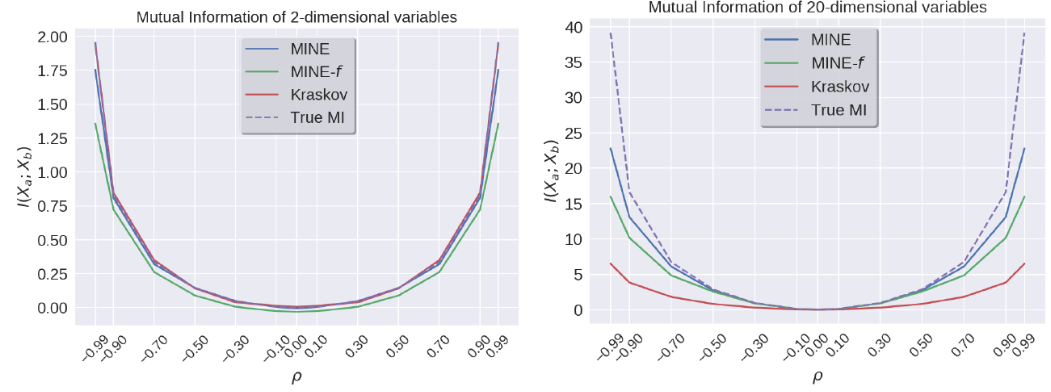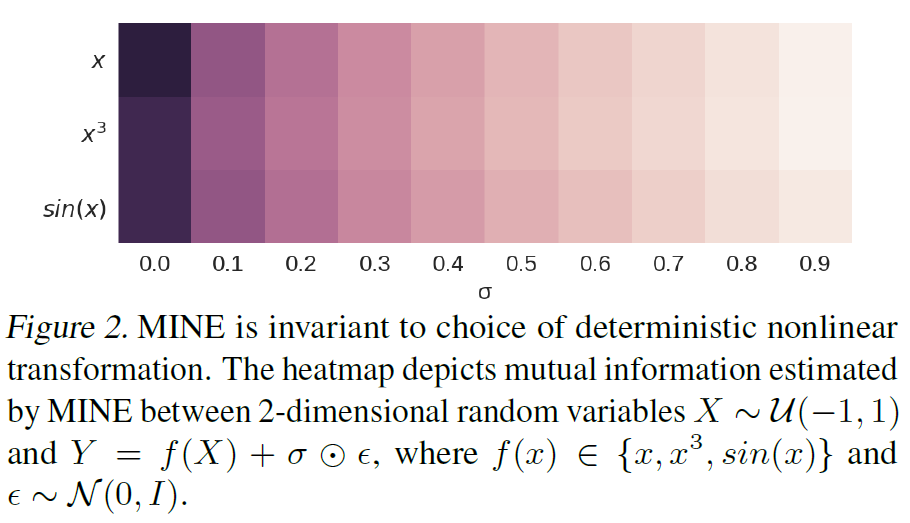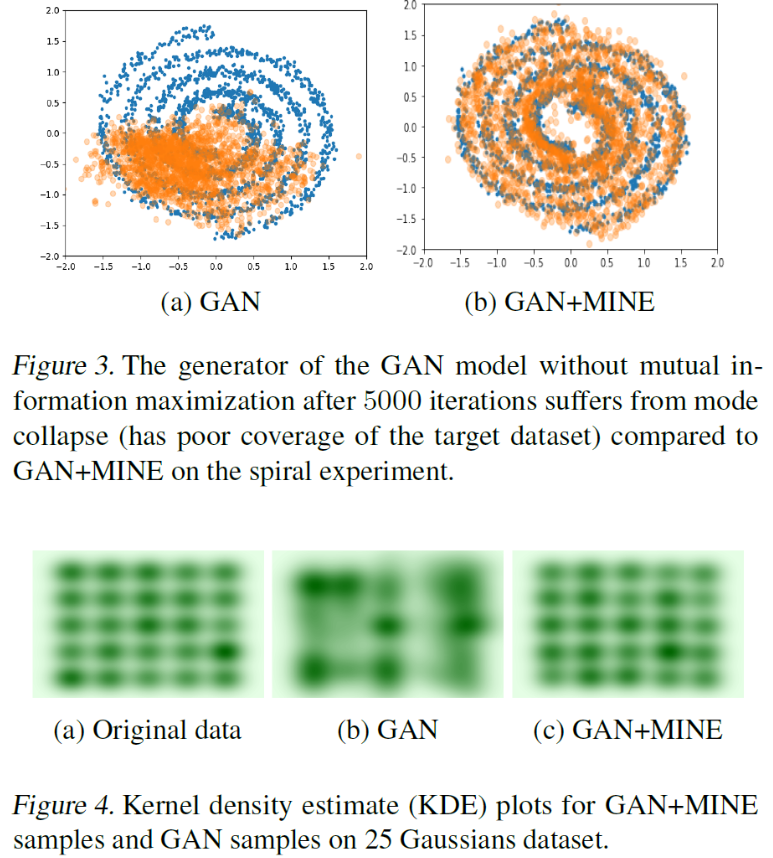## 《Mutual Information Neural Estimator》笔记

2020/12/5 10:04:53 文章标签:

1. Sketch Objective : NN to estimate mutual information : I(X,Z)DKL(PXZ∣∣PX⊗PZ)\textcolor{brown}{I(X,Z) D_{KL}(\mathbb{P}_{XZ}||\mathbb{P}_X \otimes \mathbb{P}_Z)} I(X,Z)DKL​(PXZ​∣∣PX​⊗PZ​) Approach : Donsker-Varadhan representation : DKL(P∣∣…

# 1. Sketch

Objective : NN to estimate mutual information :
I ( X , Z ) = D K L ( P X Z ∣ ∣ P X ⊗ P Z ) \textcolor{brown}{I(X,Z) = D_{KL}(\mathbb{P}_{XZ}||\mathbb{P}_X \otimes \mathbb{P}_Z)}
D K L ( P ∣ ∣ Q ) = sup ⁡ T : Ω → R E P [ T ] − log ⁡ ( E Q [ e T ] ) \textcolor{brown}{D_{KL}(\mathbb{P} || \mathbb{Q}) = \sup_{T: \Omega \rightarrow \mathbb{R}} \mathbb{E}_{\mathbb{P}}[T] - \log(\mathbb{E}_{\mathbb{Q}}[e^T])}
Mutual information neural estimator : Maximize
I ( X ; Z ) ^ n = sup ⁡ θ ∈ Θ E P X Z ( n ) [ T θ ] − log ⁡ ( E P X ( n ) ⊗ P ^ Z ( n ) [ e T θ ] ) \textcolor{brown}{\widehat{I(X;Z)}_n = \sup_{\theta \in \Theta} \mathbb{E}_{\mathbb{P}_{XZ}^{(n)}} [T_{\theta}] - \log(\mathbb{E}_{\mathbb{P}_X^{(n)} \otimes \mathbb{\hat{P}}_Z^{(n)}}[e^{T_{\theta}}])}

Q : why we use Donskere-Varadhan representation?

Transforming the NN of estimation mutual information into an optimization problem, then the gradient optimization algorithm is used to maximize the lower bound of Donskere-Varadhan representation.

# 2. AlgorithmExisting problem : SGD gradients of MINE are biased in a mini-batch setting.
G ^ B = E B [ ∇ θ T θ ] − E B [ ∇ θ T θ e T θ ] E B [ e T θ ] \textcolor{brown}{\hat{G}_B=\mathbb{E}_B[\nabla_{\theta}T_{\theta}]-\frac{\mathbb{E}_B[\nabla_{\theta}T_{\theta}e^{T_{\theta}}]}{\mathbb{E}_B[e^{T_{\theta}}]}}
Solution : replacing the estimate in the denominator by exponential moving average and a small learning rate.

# 3. Other

the author also provides another lower boundary : f-divergence representation
D K L ( P ∣ ∣ Q ) = sup ⁡ T : Ω → R E P [ T ] − E Q [ e T − 1 ] \textcolor{brown}{D_{KL}(\mathbb{P} || \mathbb{Q}) = \sup_{T: \Omega \rightarrow \mathbb{R}} \mathbb{E}_{\mathbb{P}}[T] - \mathbb{E_Q}[e^{T-1}]}
but the Donsker-Varadhan bound is stronger than f-divergence representation. the following experiments not only indicate the Donsker-Varadhan is more tighter, but also represent the lower bound-based approach is better than the non-parametric approach in mutual information estimator.correlation only reflect a linear relationship between variables. mutual information further reflect the non-linear relationship.# 4. Application

Mutual Information regularized GAN to alleviate mode-dropping.
arg ⁡ max ⁡ G E [ log ⁡ ( D ( G ( [ ϵ , c ] ) ) ) ] + β I ( G ( [ ϵ , c ] ) ; c ) \textcolor{brown}{\arg\max\limits_{G}\mathbb{E}[\log(D(G([\epsilon, c])))] + \beta I(G([\epsilon,c]);c)}
My personal understanding is that the generator learns a pattern of spoofing the discriminator, causing the generator to generate data that looks real, but only this kind. The approach here is to divide z z into two parts z = [ e , c ] z=[e,c] , and then maximize the mutual information between the generated image and c c at the same time. This corresponds to the requirement that the generated image reflect as much information about c c as possible.暂无相关的数据...